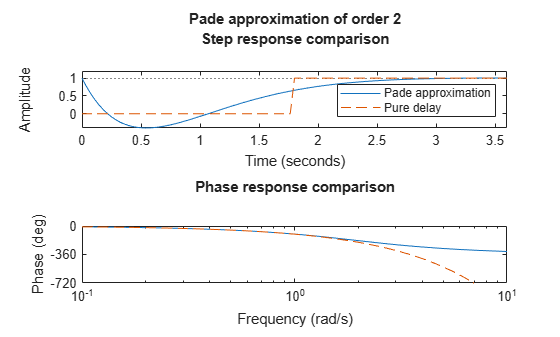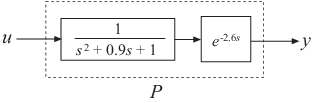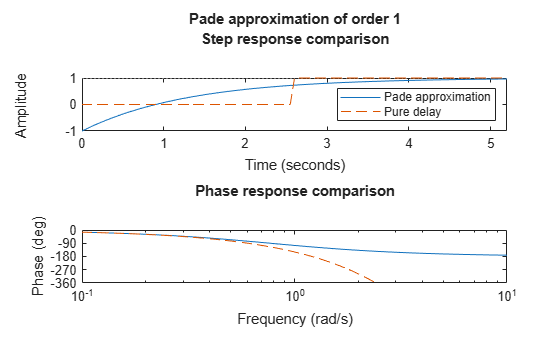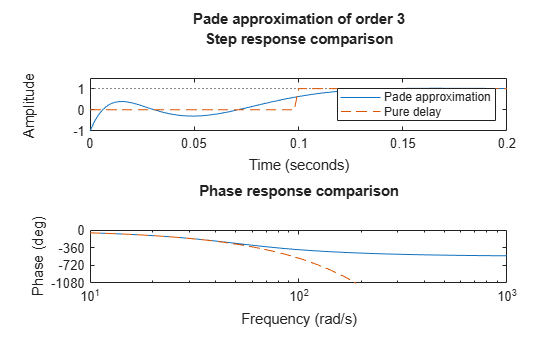Padé approximation of models with time delay

## Syntax

``[num,den] = pade(T,N)``
``pade(T,N)``
``sysx = pade(sys,N)``
``sysx = pade(sys,NU,NY,NINT)``

## Description

`pade` approximates time delays for continuous-time LTI models. Such approximations are useful to model time delay effects such as transport and computation delays within the context of continuous-time systems. The Laplace transform of a time delay of T seconds is exp(–sT). This exponential transfer function is approximated by a rational transfer function using the Padé approximation formulas from .

To approximate discrete-time models, use `absorbDelay`.

See Time Delays in Linear Systems for more information about models with time delays.

example

````[num,den] = pade(T,N)` returns the Padé approximation of order `N` of the time delay `T` in transfer function form. The output row vectors `num` and `den` contain the numerator and denominator coefficients in descending powers of s. `num` and `den` are `N`th-order polynomials.```

example

````pade(T,N)` plots the step and phase responses of the `N`th-order Padé approximation and compares them with the exact responses of the model with time delay `T`. The resultant Padé approximation has unit gain at all frequencies.```

example

````sysx = pade(sys,N)` produces a delay-free approximation `sysx` of the continuous-time delay system `sys`. All delays are replaced by their `N`th-order Padé approximation.```

example

````sysx = pade(sys,NU,NY,NINT)` specifies independent approximation orders for each input, output, and I/O or internal delay using vectors `NU`, `NY`, and `NINT`, respectively. You can use scalar values for `NU`, `NY`, or `NINT` to specify a uniform approximation order. You can also set some entries of `NU`, `NY`, or `NINT` to `Inf` to prevent approximation of the corresponding delays.```

## Examples

collapse all

For this example, compute the delay-free numerator and denominator coefficients for a time delay of 1.8 seconds for a second-order system.

```T = 1.8; N = 2; [num,den] = pade(T,N)```
```num = 1×3 1.0000 -3.3333 3.7037 ```
```den = 1×3 1.0000 3.3333 3.7037 ```

You can also plot the step and phase response of the delay-free approximation and the original system with time delay. Use the `pade` command without output arguments to generate the comparison plots.

`pade(T,N)`For this example, find the Padé approximation of the following continuous-time open loop system.Create the open-loop system with an output delay.

```s = tf('s'); T = 2.6; sys = exp(-T*s)/(s^2+0.9*s+1)```
```sys = 1 exp(-2.6*s) * --------------- s^2 + 0.9 s + 1 Continuous-time transfer function. ```

`sys` is a second-order transfer function (`tf`) object with a time delay.

Next, compute the first-order Padé approximation of `sys`.

`sysx = pade(sys,1)`
```sysx = -s + 0.7692 ---------------------------------- s^3 + 1.669 s^2 + 1.692 s + 0.7692 Continuous-time transfer function. ```

`pade` replaces all time delays in `sys` with a first-order approximation. Therefore, `sysx` is a third-order transfer function with no delays.

You can also plot and compare the step and phase response of the delay-free approximated model and the model with time-delay. Use the time delay and order values to create the plot.

`pade(T,1)`Compute a third-order Padé approximation of a 0.1-second I/O delay.

```s = tf('s'); sys = exp(-0.1*s); sysx = pade(sys,3)```
```sysx = -s^3 + 120 s^2 - 6000 s + 1.2e05 -------------------------------- s^3 + 120 s^2 + 6000 s + 1.2e05 Continuous-time transfer function. ```

Here, `sys` is a dynamic system representation of the exact time delay of 0.1 s. `sysx` is a transfer function that approximates that delay.

Compare the time and frequency responses of the true delay and its approximation. Calling the `pade` command without output arguments generates the comparison plots. In this case the first argument to `pade` is just the magnitude of the exact time delay, rather than a dynamic system representing the time delay.

`pade(0.1,3)`For this example, consider the MIMO state-space model `sys` contained in `ssModel.mat`. `sys` has two inputs and three outputs, and contains input, output and internal delays.

Load the data and examine `sys`.

```load('ssModel.mat','sys') sys```
```sys = A = x1 x2 x1 2.893 1.497 x2 -0.1138 -0.5279 B = u1 u2 x1 -1.334 -4.155 x2 1.127 -0.06161 C = x1 x2 y1 -2.416 -0.6317 y2 1.688 1.063 y3 3.509 1.84 D = u1 u2 y1 1.019 1.999 y2 0 -3.658 y3 0 -5.885 (values computed with all internal delays set to zero) Input delays (seconds): 1.5 0.3 Output delays (seconds): 0.2 0.8 1.3 Internal delays (seconds): 2.1 1.3 Continuous-time state-space model. ```

Specify the approximation orders for the input, output, and internal delays, and compute the Padé approximation. Set approximation orders to `Inf` to prevent approximation of the corresponding delays.

```NU = [3 Inf]; NY = [1 Inf 2]; NINT = [Inf 2]; sysx = pade(sys,NU,NY,NINT)```
```sysx = A = x1 x2 x3 x4 x5 x6 x7 x1 -10 0 0 -9.665 -2.527 -7.305 0 x2 0 -4.615 -3.55 14.04 7.358 21.51 0 x3 0 2 0 0 0 0 0 x4 0 0 0 2.893 1.497 4.115 0 x5 0 0 0 -0.1138 -0.5279 -0.2169 0 x6 0 0 0 -8.011 -3.193 -4.615 -3.55 x7 0 0 0 0 0 2 0 x8 0 0 0 0 0 0 0 x9 0 0 0 0 0 0 0 x10 0 0 0 0 0 0 0 x8 x9 x10 x1 16.3 0 4.527 x2 0 0 0 x3 0 0 0 x4 -5.335 0 -1.482 x5 4.51 0 1.253 x6 0 0 0 x7 0 0 0 x8 -8 -6.667 -2.222 x9 4 0 0 x10 0 4 0 B = u1 u2 x1 -4.075 7.996 x2 0 -23.54 x3 0 0 x4 1.334 -4.155 x5 -1.127 -0.06161 x6 0 10.1 x7 0 0 x8 4 0 x9 0 0 x10 0 0 C = x1 x2 x3 x4 x5 x6 x7 x8 y1 5 0 0 2.416 0.6317 1.826 0 -4.075 y2 0 0 0 1.688 1.063 3.074 0 0 y3 0 -2.308 0 3.509 1.84 5.377 0 0 x9 x10 y1 0 -1.132 y2 0 0 y3 0 0 D = u1 u2 y1 1.019 -1.999 y2 0 -3.658 y3 0 -5.885 (values computed with all internal delays set to zero) Input delays (seconds): 0 0.3 Output delays (seconds): 0 0.8 0 Internal delays (seconds): 2.1 Continuous-time state-space model. ```

The resultant approximation `sysx` still has specific input, output, and internal delays where the corresponding approximation orders are `Inf`.

## Input Arguments

collapse all

Time delay value, specified as a positive scalar. The Padé approximation of a zero delay (`T = 0`) is always unit gain.

Desired order of the continuous-time approximation model, specified as a positive integer.

Dynamic system with time delay, specified as a SISO or MIMO dynamic system model. Dynamic systems that you can use include:

For discrete-time models, use `absorbDelay`.

Approximation orders for the input channel, specified as

• A scalar, to use the same approximation order across all the inputs.

• A vector, to specify individual approximation order values per input. You can use `Inf` for specific inputs to prevent approximation of corresponding delays.

Approximation orders for the output channel, specified as

• A scalar, to use the same approximation order across all the outputs.

• A vector, to specify individual approximation order values per output. You can use `Inf` for specific outputs to prevent approximation of corresponding delays.

Approximation orders for I/O delay (transfer functions or zero-pole-gain models) or internal delay (state-space models), specified as

• A scalar, to use the same approximation order across all the I/O or internal delays.

• A vector, to specify individual approximation order values per I/O or internal delay. You can use `Inf` for specific I/Os to prevent approximation of corresponding delays.

## Output Arguments

collapse all

Numerator coefficients of delay-free transfer function, returned as a row vector.

Denominator coefficients of delay-free transfer function, returned as a row vector.

Padé approximated system, returned as a model object of the same type as `sys`.

## Limitations

• Padé approximation is valid only at low frequencies and provides better frequency-domain approximation than time-domain approximation. Therefore, compare the true and approximate responses to choose the right approximation order and check the approximation validity.

• High-order Padé approximations produce transfer functions with clustered poles. Because such pole configurations tend to be very sensitive to perturbations, avoid Padé approximations with order `N>10`.

 Golub, Gene H., and Charles F. Van Loan. Matrix Computations. 2nd ed. Johns Hopkins Series in the Mathematical Sciences 3. Baltimore, Md: Johns Hopkins University Press, 1989. pp. 557-558.